# KSEEB Solutions for Class 9 Maths Chapter 5 Triangles Ex 5.5

KSEEB Solutions for Class 9 Maths Chapter 5 Triangles Ex 5.5 are part of KSEEB Solutions for Class 9 Maths. Here we have given Karnataka Board Class 9 Maths Chapter 5 Triangles Exercise 5.5.

## Karnataka Board Class 9 Maths Chapter 5 Triangles Ex 5.5

Question 1.
ABC is a triangle. Locate a point in the interior of ∆ABC which is equidistant from all the vertices of ∆ ABC.
Solution:
ABC is a triangle. To locate a point in the interior of ∆ABC which is equidistant from all the vertices of ∆ABC we have to find out circumcentre means the point where three perpendicular bisectors meet.
E.g. Three sides of ∆ABC are,
AB = 5 cm, BC = 4 cm, and AC = 6 cm.‘S’ is the circumcentre of ∆ABC

Question 2.
In a triangle locate a point in its interior which is equidistant from all the sides of the triangle.
Solution:
Incentre is equidistant from three sides of a ∆. This is at the point where angular bisectors meet. This is called T.
E.g. In ∆ABC, AB = 6 cm, ∠B = 100°, ∠A = 50°.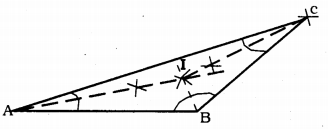Point T is equidistant from three sides.

Question 3.
In a huge park, people are concentrated at three points
A: where there are different slides and swings for children,
B: near which a man-made lake is situated,
C: which is near to a large parking and exit.
Where should an icecream parlour be set up so that the maximum number of persons can approach it?
(Hint: The parlour should be equidistant from A, B, and C).
Solution:
A: In a park where there are different slides and swings for children.
B: near which a man-made lake is situated.
C: which is near to a large parking and exit.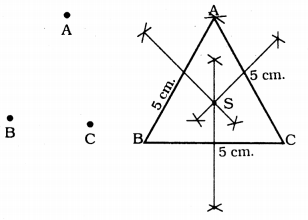In ∆ABC, to locate a point equidistant from three sides, we have to find out perpendicular bisectors, which means where all perpendicular bisectors meet. This is called S’.
AB = BC = CA = 5 cm.
∴ The ice cream shop is at ‘S’.

Question 4.
Complete the hexagonal and star-shaped Rangolies by filling them with as many equilateral triangles of side 1 cm as you can. Count the number of triangles in each case. Which has more triangles?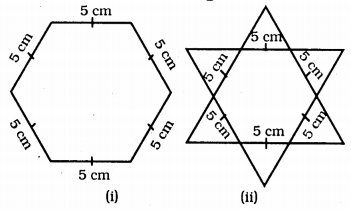Solution:
Fig. (i): Regular Hexagonal with side 5 cm. is constructed. It has 6 equal sides.
Measure of each side is 5 cm.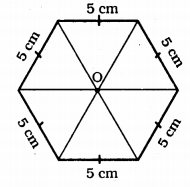Number of equilateral ∆ with 1 cm side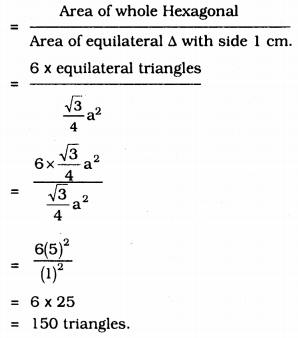In Fig. (ii) : there are 12 equilateral triangles with side 5 cm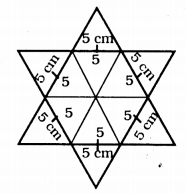∴ Number of equilateral A with 1 cm side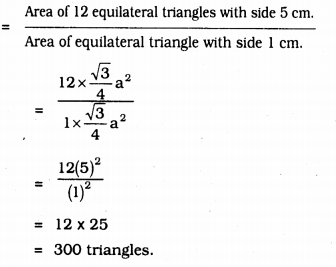We hope the KSEEB Solutions for Class 9 Maths Chapter 5 Triangles Ex 5.5 help you. If you have any query regarding Karnataka Board Class 9 Maths Chapter 5 Triangles Exercise 5.5, drop a comment below and we will get back to you at the earliest.

error: Content is protected !!